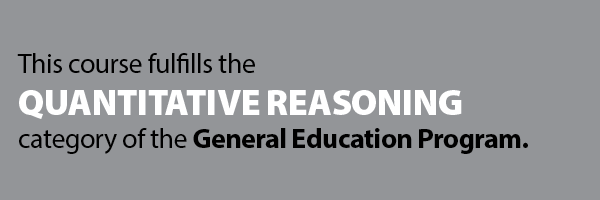Jul 21, 2019
 HELP 2018-2019 Undergraduate Catalog THIS IS NOT THE CURRENT CATALOG. LINKS AND CONTENT ARE OUT OF DATE!Print-Friendly Page[Add to Portfolio]

# MATH 120 - Calculus I [GEQR]

Calculus of functions of a single variable; differential calculus, including limits, derivatives, techniques of differentiation, the mean value theorem and applications of differentiation to graphing, optimization and rates. Integral calculus, including indefinite integrals, the definite integral, the fundamental theorem of integral calculus, and applications of integration to area and volume.

Credit 4 hrs May not be repeated for additional credit
Grade Mode Normal (A-F) Course Rotation Fall, Winter, and Summer

Prerequisite(s) placement or (at least a C in any of the following group of courses: (MATH 105  and MATH 107 ) or (MATH 112 ) or MATH 210  and MATH 107 ))Notes -